# CLASS 11 MATHS CHAPTER-10 STRAIGHT LINES

### Exercise 10.1

Question1. Draw a quadrilateral in the Cartesian plane, whose vertices are (– 4, 5), (0, 7),(5, – 5) and (– 4, –2). Also, find its area.

Solution :
Let ABCD be the given quadrilateral with vertices A (–4, 5), B (0, 7), C (5, –5), and D (–4, –2).

Then, by plotting A, B, C, and D on the Cartesian plane and joining AB, BC, CD, and DA, the given quadrilateral can be drawn as

= To find the area of quadrilateral ABCD, we draw one diagonal, say AC.

Accordingly, area (ABCD) = area (ΔABC) + area (ΔACD)

We know that the area of a triangle whose vertices are (x1, y1), (x2, y2), and (x3, y3) is

Question2. The base of an equilateral triangle with side 2a lies along the y-axis such that the mid-point of the base is at the origin. Find vertices of the triangle.

Solution :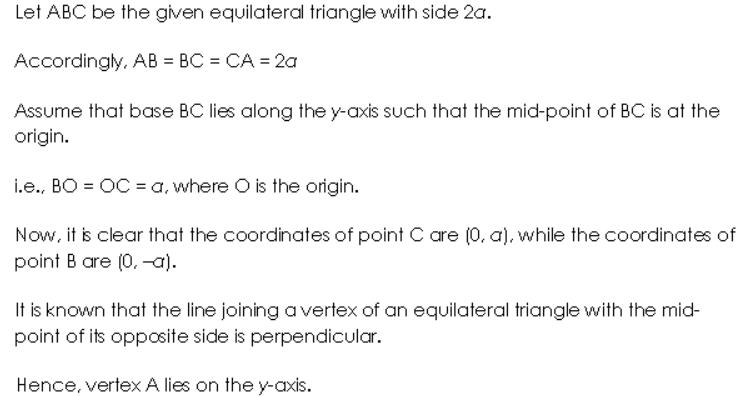.

Question3. Find the distance between P(x,y1) and Q(x2 ,y2) when :

(i) PQ is parallel to the y-axis,

(ii) PQ is parallel to the x-axis.

Solution :Here, the points are P(x1,y1) and Q(x2,y2).

When PQ is parallel to the y -axis, we have x1 = x2

The distance between P and Q is,

√((x2−x1)2+(y2−y1)2)

= √(y2−y1)(since x1 = x2.)

=|y2−y1|

Therefore, the distance between P(x1,y1)
and Q(x2,y2) when PQ
is parallel to the y

axis is |y2−y1|
.

(ii) PQ is parallel to x – axis, then

The points are P(x1,y1) and Q(x2,y2) When PQ is parallel to the x-axis, we know y1=y2
Distance between P and Qis

Therefore, the distance between P(x1,y1) and Q(x2,y2) when PQ is parallel to the x axis is |x2−x1|

Question4. Find a point on the x-axis, which is equidistant from the points (7, 6) and (3, 4).

Solution :Let A(7,6) and B(3,4) be the given points.
Assume C(a,0) as the point on the X – axis that is equidistance from the points (7,6)
and (3,4).In general, Distance between two points is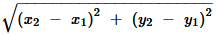And PR = .Now, Distance between A and C = Distance between B and C

Question5. Find the slope of a line, which passes through the origin, and the mid-point of the line segment joining the points P (0, – 4) and B (8, 0)

Solution :The coordinates of the midpoint of the line segment joining two points are,

(x1+x2 / 2  , y1+y2  / 2)

Then, the coordinates of the mid-point of the line segment joining the points P(0,−4)
and B(8,0)

are,

(0+8 / 2,− 4+0 / 2) = (4,−2)

We know, the slope (m)
of a non-vertical line passing through the points (x1,y1) and (x2,y2)

is,

m= y2−y1 /  x2−x1,

where

x2 ≠ x1

Thus, the slope of the line passing through (0,0,
, and (4,−2)

is,

⇒−2−04−0

=−24

=−12

Therefore, the slope of a line, which passes through the origin, and the mid-point of the

segment joining the points P(0,−4)
and B(8,0) is −12.
.

Question6. Without using the Pythagoras theorem, show that the points (4, 4), (3, 5) and (–1, –1) are the vertices of a right angled triangle.

Solution :
The vertices of the given triangle are P(4,4),Q(3,5), and R(−1,−1).

We know, the slope (m) of a non-vertical line passing through the points (x1,y1) and (x2,y2) is,

Question7. Find the slope of the line, which makes an angle of 30° with the positive direction of y-axis measured anticlockwise.

Solution :Given that, the line makes an angle of 30 with the positive direction of y -axis

measured anticlockwise.

Plot the figure,

From the figure, the angle made by the line with the positive direction of the x-axis

measured anticlockwise is,

90+30=120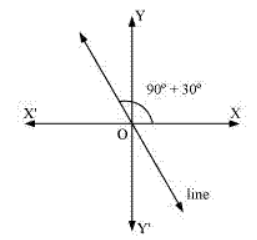Now, the slope of the given line is tan120

Rewritetan120as tan(180−60)

=−tan60

=−√3

Therefore, the slope of the line, which makes an angle of 30∘
with the positive direction of y-axis measured anticlockwise is −√3

Question8. Find the value of x for which the points (x, – 1), (2,1) and (4, 5) are collinear.

Solution :.Given that, the points are (x,−1),(2,1) and (4,5)

If points P(x,−1),Q(2,1) and R(4,5) are collinear, then Slope of PQ= Slope of QR

⇒(1−(−1)) / (2−x) = (5−1) / (4−2) Open bracket and simplify,

⇒(1+1)  / (2−x) = 42

⇒2 / (2−x) = 2

⇒2=4−2x

⇒2x=2

⇒x=1

Therefore, the value of x for which the points (x,−1),(2,1) and (4,5) are collinear is 1.

Question9. Without using distance formula, show that points (– 2, – 1), (4, 0), (3, 3) and (–3, 2) are the vertices of a parallelogram.

Solution :
Let points (−2,−1),(4,0),(3,3) and (−3,2) be respectively denoted by P,Q,R and S.

Question10. Find the angle between the x-axis and the line joining the points (3,–1) and (4,–2).

Solution :
Let A (3,–1) and B (4,–2) be two points. Let Q be the angle which AB makes with positive direction of axis.

The points are (3, −1) and (4, −2).

The slope (m) of a non-vertical line passing through the points (x1,y1) and (x2,y2) is,

m= (y2−y1)  / ( x2−x1),where x2 ≠ xThe slope of the line joining the points (3,−1) and (4,−2)is,

m=−2−(−1)  / 4−3

=−2+1

=−1
The inclination (θ) of the line joining the points (3,−1) and (4,−2) is,
tanθ = m

Substitute the value of m
tanθ = −1
⇒ θ = (90+45)

= 135

Therefore, the angle between the x-axis and the line joining the points (3,−1)  and (4,−2)
is 135

Question11. The slope of a line is double of the slope of the another line. If tangent of the angle between them is 1/3 find the slopes of the lines.

Solution :Take m,m1 as the slopes of the two lines.
Given that, slope of a line is double of the slope of another line.
That is, m1=2m If θ is the angle between the lines l1 and l2 with slopes m1 and m2
We have,

Question12. A line passes through (x1,y1) and (h,k) .If slope of the line is m, show that k−y= m(h−x1)

Solution :.Given that, the slope of the line is m

The slope of the line passing through (x1, y1) and (h,k) is k−y1 / h−x1

.Now,
k−y1 / h−x1=m

⇒k−y1 = m(h−x1)

Therefore, if the line passes through (x1,y1)
and (h,k) with a slope of the m

,

k−y=  m(h−x1)

Question13. If three points (h, 0), (a, b) and (0, k) lie on a line, show that

Solution :The points P(h,0),Q(a,b), and R(0,k)

lie on a line. Slope of PQ= Slope of QR

Cross multiply,

⇒−ab=(k−b)(a−h) Open brackets,

⇒−ab=ka−kh−ab+bh

⇒ka+bh=kh

Divide both sides by kh
,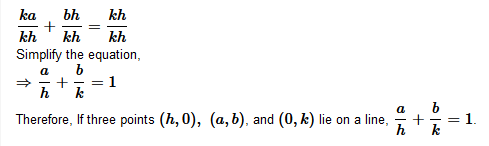Question14. Consider the following population and year graph, find the slope of the line AB and using it, find what will be the population in the year 2010?

Solution :
Given: The points on the line are A (1985, 92) and B (1995, 97).

⇒ Slope of AB = (97 – 92) / (1995 -1985)

⇒ 5/10

⇒ 1/2

LAssume y as the population in the year 2010. Then, according to the given graph, line  AB must pass through the point C(2010,y)
∴ Slope of AB= Slope of BC

Since points A, B and C lie on the line.

### In Exercises 1 to 8, find the equations of the line which satisfy the given conditions:

Question1. Write the equations for the x-and y-axes.

Solution :
The y-coordinate of every point on the x-axis is 0.

Therefore, the equation of the x-axis is y = 0.

The x-coordinate of every point on the y-axis is 0.

Therefore, the equation of the y-axis is x = 0.

Question2. Passing through the point (– 4, 3) with slope 1/2

Solution :Given that, the point (−4,3) and slope of the line is 1 / 2.

The equation of the line passing through point (x0,y0), whose slope is m, is

(y−y0)=m(x−x0).

Thus, the equation of the line passing through point (−4,3), whose slope is 1 / 2, is (y−3) = 1 / 2 (x+4)

Cross multiply,

2(y−3)=x+4

2y−6=x+4

Move the constants together,
x−2y+10=0

Therefore, the equation of the line which passes through the point (−4,3)
with slope 12 is x−2y+10=0.

Question3. Passing through (0, 0) with slope m.

Solution :Given that, the point is  (0,0) and slope is m.

The equation of the line passing through point (x0,y0) , whose slope is m, is

(y−y0) = m(x−x0).
Substitute the values in the equation,

⇒(y−0) = m(x−0)

⇒y = mx

Therefore, the equation of the line which passes through (0,0) with slope m
is y = mx.

Question4. Passing through (2,2√3) and inclined with the x-axis at an angle of 75

Solution :Given that, the point is  (2,2√3)

The slope of the line that inclines with the x-axis at an angle of 75

That is, m = tan75

m = tan (45 + 45)

The equation of the line passing through point (x0,y0), whose slope is m, is (y−y0) = m(x−x0).
Thus, if a line passes through (2,2√3) and inclines with the x-axis at an angle of 75,then the equation of the line is,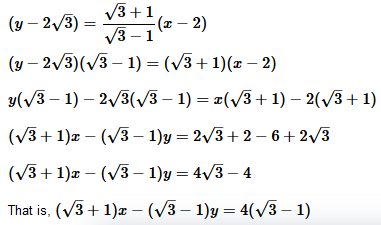Therefore, the equation of the line which passes through (2,2√3) and is inclined with the x-axis at an angle of 75∘is (√3+1)x−(√3−1)y = 4(√3−1).

Question5: Intersecting the x-axis at a distance of 3 units to the left of origin with slope –2.

Solution :
It is known that if a line with slope m makes x-intercept d, then the equation of the line is given as

y = m(x – d)

For the line intersecting the x-axis at a distance of 3 units to the left of the origin, d = –3.

The slope of the line is given as m = –2

Thus, the required equation of the given line is

y = –2 [x – (–3)]

y = –2x – 6

i.e., 2x + y + 6 = 0

Question6. Intersecting the y-axis at a distance of 2 units above the origin and making an angle of 30with positive direction of the x-axis.

Solution :Given, the line intersects the y-axis at a distance of 2
units above the Origin.The line makes an angle of 30∘ with the positive direction of the x-axis.
That is, c=2

m=tan30

=–√13

If a line with slope  makes y – intercept c, then the equation of the line is,

y=mx+c

Substitute the values,

Therefore, the equation of the line which intersects the y-axis at a distance of 2
units above the origin and makes an angle of 30 with the positive direction of the x-axis is x−√3y+ √23 =0

Question7. Passing through the points (–1, 1) and (2, – 4).

Solution :

Given that the line makes angle 30 with positive direction of y- axis.

Thus, the angle made by line with x axis is,

90+30 = 120∘

Slope m = tanθ

=tan120

=−tan60∘

= √3

Therefore, the slope of the line, which makes an angle of 30∘
with the positive direction of y-axis measured anticlockwise is −√3

Question8. Perpendicular distance from the origin is 5 units and the angle made by the perpendicular with the positive x-axis is 30.

Solution :.Let p be the length of the normal from the origin to a line and ω be the angle made by the normal with the positive direction of the x-axis

Now, equation of the line is xcosω+ysinω=p

Here, p=5 units and ω=30

Thus, the equation of the given line is,

xcos30 + ysin30 =5

Substitute the values,

x√3 / 2 + y⋅1 / 2 = 5

That is, √3 x + y = 10

Therefore, the equation of the line which is at a perpendicular distance of 5
units from the origin and the angle made by the perpendicular with the positive x-axis is 30∘is √3x + y = 10.

Question9. The vertices of ∆ PQR are P (2, 1), Q (–2, 3) and R (4, 5). Find equation of the median through the vertex R.

Solution :Given that, the vertices of ΔPQR are P(2,1),Q(−2,3) and R(4,5)

Let RL
be the median through vertex R

And L be the mid-point of PQ

By mid-point formula, the coordinates of point L
are,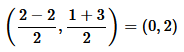Equation of required median RS is

Question10. Find the equation of the line passing through (–3, 5) and perpendicular to the line through the points (2, 5) and (–3, 6).

Solution :The slope of the line joining the points (2,5) and (−3,6)

is,

m= 6−5 / −3−2

= 1 / −5

It is known that two non-vertical lines are perpendicular to each other if and only if their slopes are negative reciprocals of each other.

Thus, slope of the line perpendicular to the line through the points (2,5)
and (−3,6)

is,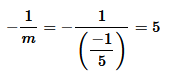The equation of the line passing through point (−3,5)
, whose slope is5

is,

(y−5) = 5(x+3)

Expand brackets,

y−5 = 5x+15

That is, 5x−y+20=0

Therefore, the equation of the line passing through (−3,5)
and perpendicular to the line through the points (2,5) and (−3,6) is 5x−y+20=0.

Question11. A line perpendicular to the line segment joining the points (1, 0) and (2, 3) divides it in the ratio 1: n. Find the equation of the line.

Solution :By section formula, the coordinates of the point that divides the line segment joining the points (1,0) and (2,3) in the ration 1:n

is,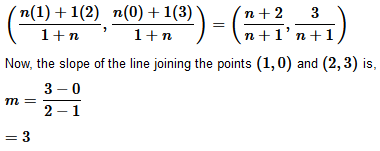It is known that two non-vertical lines are perpendicular to each other if and only if their slopes are negative reciprocals of each other.

Thus, slope of the line that is perpendicular to the line joining the points (1,0)
and (2,3)

is,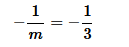The equation of the line passing through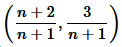and whose slope is −1 / 3

is ,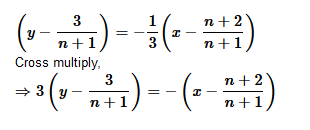Therefore, equation of the line perpendicular to the line segment joining the points (1,0)
and (2,3) divides it in the ration 1:n is (1+n)x+3(1+n)y=n+11.

Question12. Find the equation of the line cuts off equal intercepts on the coordinate axis and passes through the point (2, 3).

Solution :The equation of a line in the intercept form is xa + yb =1

Here,  a andb
are the intercepts on x and y axes respectively.

Given that,  the line cuts off equal intercepts on both the axes.

That is, a=b

.

Now, xa + ya = 1

⇒ x + y = a

The given line passes through the point (2,3)

, this equation reduces to

2 + 3 = a

⇒a=5

Substitute the value of a
in x + y =  a

That is, x + y = 5

Therefore, the equation of a line that cuts off equal intercepts on the coordinate axes

and passes through the points (2,3)
is x + y = 5.

Question13. Find the equation of the line passing through the point (2, 2) and cutting off intercept on the axis whose sum is 9.

Solution :
Given: Line passes through point (2, 2).  a = 6 , and b = 9-6 = 3 then the equation of the line is

x/ 6 + y/3 = 1 ⇒ x + 2y – 6 = 0

if a = 3 and b = 9-3  = 6  , then the equation  of the line is

x/3 + y/6 = 1 ⇒ 2x + y -6 = 0

the equatiuon  of a line in the intercept form is  x/a +y/b = 1  — (1)

Question14. Find equation of the line through the point (0, 2) making an angle 2π/3 with the positive x-axis.

Also, find the equation of line parallel to it and crossing the y-axis at a distance of 2 units below the origin.

Solution :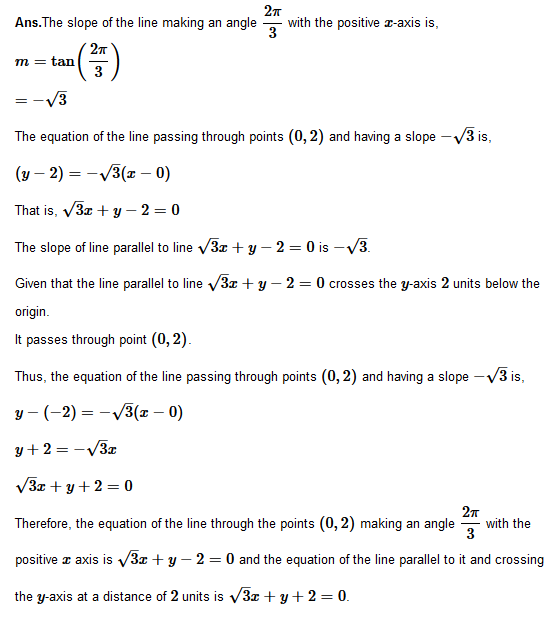Question15. The perpendicular from the origin to a line meets it at the point (–2, 9), find the equation of the line.

Solution :Given that, The perpendicular from the origin to a line meets it at the point (−2,9)

.

The slope of the line joining the origin (0,0)
and point (−2,9)

is,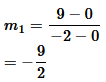Then, the slope of the line perpendicular to the line joining the origin and points (−2,9)

is,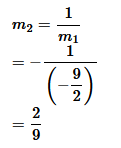Now, the equation of the line passing through point (−2,9)
and having a slope m2 is,(y−9)=2/ 9(x+2)

Cross multiply and expand brackets,

9y−81 = 2x+4

That is, 2x−9y+85=0

Therefore, the equation of the line is 2x−9y+85=0
.

Question16. The length L (in centimeter) of a copper rod is a linear function of its Celsius temperature C. In an experiment if L = 124.942 when C = 20 and L = 125.134 when C = 110, express L in terms of C.

Solution :It is given that when C=20,  L=124.942 and when C=110,  L=125.134

The points (20,124.942)
and (110,125.134) satisfy the linear relation between Land C.

Assume C
along the x
-axis and L along the y
-axis, we have two points, (20,124.942)

and (110,125.134)
in the XY

plane.

Thus, the linear relation between L
and C

is the equation of the line passing through

thepoints (20,124.342)
and (110,125.134)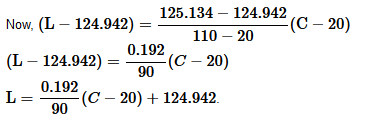Question17. The owner of a milk store finds that he can sell 980 liters of milk each week at Rs. 14 litre and 1220 liters of milk each week at Rs. 16 liters. Assuming a linear relationship between selling price and demand, how many liters could he sell weekly at Rs. 17 liter?

Solution :Given that, the owner can sell 980
liters of milk each week at Rs 14/ liter and 1220
liters of milk each week at Rs 16/

liter.

The relationship between the selling price and demand is linear.

Assume selling price per liter along the x
-axis and demand along the y
-axis, we have two points (14,980) and (16,1220) in the XY

plane that satisfy the linear relationship between selling price and demand.

Thus, the line passing through points (14,980)
and (16,1220)

That is, y−980=((1220−980) / (16−14)) (x−14)

y−980=(240 / 2) (x−14)

y−980=120(x−14)

y=120(x−14)+980

If x= Rs 17 / liter,

y=120(17−14) + 980

⇒y = 120×3 + 980

=360 + 980

=1340

Therefore, the owner of the milk store could sell 1340
liters of milk weekly at Rs 17/liter

Question18. P(a , b) is the mid-point of a line segment between axis. Show that equation of the line is

Solution :

Then

Question19. Point R (h, k) divides a line segment between the axes in the ratio 1: 2. Find bequation of the line.

Solution :
Let AB and BA be two points where the line intersect p (a,b)and axis respectively and R is a point divides AB in the ratio 1: 2.

Question20. By using the concept of equation of a line, prove that the three points (3, 0),(– 2, – 2) and (8, 2) are collinear.

Solution :It is necessary to show that the line passing through points (3,0) and (−2,−2) also passes through point (8,2)in order to show that the points (3,0),(−2,−2) and (8,2)

are collinear
The equation of the line passing through points (3,0)
and (−2,−2) is,

.Thus, the line passing through points (3,0) and (−2,−2) also passes through point (8,2)

.Therefore, the points (3,0),(−2,−2)
, and (8,2) are collinear.

### Exercise 10.3

Question1. Reduce the following equations into slope-intercept form and find their slopes and the intercepts.

(i) x + 7y = 0,

(ii) 6x + 3y – 5 = 0,

(iii) y = 0.

Solution :
(i) Given: x +7y = 0

it can be writen as

⇒ y =- 1/7x + 0 ——(1)

Therefore, the above equation is of the form y = mx + c, where m =−(1 / 7)

(ii) Given: 6x + 3y – 5 = 0,

Given that, the equation is 6x+3y−5=0
Slope – intercept form is represented as y=mx+c
, where m is the slope and c is the y

intercept.

Now, the equation can be expressed as,

3y=−6x+5

y=−6/3x+5/3

=−2x+5/3

Therefore, the above equation is of the form y=mx+c
, where m=−2 and c=5/3.

(iii) Given:  y = 0

Given that, the equation is y=0

Slope – intercept form is given by y=mx+c
, where m is the slope and c

is the y intercept.

Then, y=0 × x + 0

Therefore, the above equation is of the form y=mx+c
, where m=0 and c=0.

Question2. Reduce the following equations into intercept form and find their intercepts on the axis:

(i) 3x + 2y – 12 = 0,

(ii) 4x – 3y = 6,

(iii) 3y + 2 = 0.

Solution :
(i) Given: 3x + 2y – 12 = 0,

it can be writen as

3x + 2y = 12

3x/12 + 2y/12 = 1—————– (1)

the equation  is of the form  x/a + y/b = 1 Where a = 4 and b = 6 .

Therefore equation  (1) is in  intercept from the intercepts on the x and y axes are 4 and 6

(ii) Given: 4x -3y = 6

Divided  both side by 6

(iii) Given: 3y +2 = 0

iven that, the equation is 3y + 2 = 0

Rewrite the equation,

3y =−2

Divide both sides by −2

G⇒y(−2/3)=1

Now, equation is in the form x/a+y/b = 1
, where a=0 and b=−2/3

.

Therefore, the equation is in the intercept form, where the intercept on the y-axis is −23 and it has no intercept on the x-axis.

Question3. Reduce the following equations into normal form. Find their perpendicular distances from the origin and angle between perpendicular x-axis:

(i) x−√3y + 8=0,

(ii) y – 2 = 0,

(iii) x – y = 4.

Solution :
(i) Given: x−√3y + 8=0,

(ii)

Given that, the equation is y−2=0

This can be reduced as,

0.x+1.y=2
Divide both sides by 02+12−−−−−−√=1, we get
0.x+1.y=2
⇒xcos90+ysin90 = 2
This equation is in the normal form.

When comparing the equation with the normal form of equation of line

xcosω+ysinω = p
, we get ω = 90 and p=2

Therefore, the perpendicular distance of the line from the origin is 2
, while the angle between the perpendicular and the positive x-axis is 90∘.

(iii) Given:  x−y=4

Ans.Given that, the equation is x−y=4

This can be reduced as 1.x+(−1)y=4

Divide both sides by

Question 4. Find the distance of the point (–1, 1) from the line 12 (x + 6) = 5(y – 2).

Solution :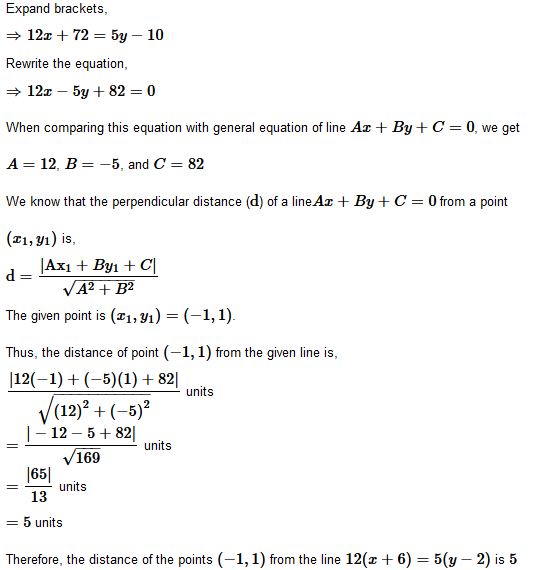5. Find the points on the x-axis, whose distances from the line x/3 + y/4=1 are 4 units.

Solution :
Let  can be write as 4x+3y−12=0

When comparing this equation with general equation of line Ax+By+C=0

, we get

A=4,B=3, and C=−12

Let (a,0)
be the point on the x-axis whose distance from the given line is 4

units.

We know that the perpendicular distance (d) of a line Ax+By+C=0 from a point

Question6. Find the distance between parallel lines

(i) 15x + 8y – 34 = 0 and 15x + 8y + 31 = 0

(ii) l (x + y) + p = 0 and l (x + y) – r = 0.

Solution :
(i) We know that the distance(d) between parallel lines Ax+By+C1=0 and
Ax+By+C2=0 is,

The given parallel lines are 15x+8y−34 = 0 and 15x+8y+31 = 0

Here, A= 15,B = 8,C1 = −34, and C2 =31
Thus, the distance between the parallel lines is,

Question7.  Find equation of the line parallel to the line 3x − 4y + 2=0 and passing through the point (–2, 3).

Solution :Here, the equation of the given line is 3x−4y+2=0
Rewrite as,
y=3x/4 + 24
This can be write as,

y=34x + 12 , which is of the form y=mx+c

Now, slope of the given line = 34

It is known that parallel lines have the same slope.

Thus, slope of the other line is m=34

Now, the equation of the line which has a slope of 34

and passes through the points

(−2,3)

is,

(y−3)=3/4 {x−(−2)}

Cross multiply and expand brackets,

4y−12 = 3x+6

Rewrite as,

3x−4y+18=0

Therefore, the equation of the line parallel to the line 3x−4y+2=0

and passing

through the point (−2,3)
is 3x−4y+18=0.

Question8. Find equation of the line perpendicular to the line x – 7y + 5 = 0 and having x intercept 3.

Solution :
Given:The given equation of the line is x−7y+5=0

It can be write as,

y = 17x + 57  which is of the form y = mx + c

Now, Slope of the given line  = 17
The slope of the line perpendicular to the line having a slope of 17 is,

m=−1/(1/7)=−7

The equation of the line with slope −7 and x-intercept 3 is,
y=m(x−d)

Substitute the values,

⇒y=−7(x−3)

Expand bracket,

⇒y = −7x + 21

⇒ 7x + y =21

Therefore, the equation of the line perpendicular to the line x−7y+5=0
and having x

intercept 3 is 7x+y=21.

through the point (−2,3) is 3x−4y+18=0.

Question9. Find angles between the lines √3x + y = 1 and x + √3y =1.

Solution :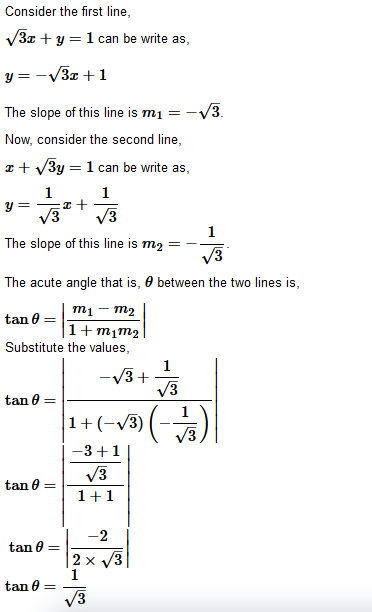θ = 30
Thus, the angle between the lines √3x + y = 1
and x+√3y=1 is either 30

or

180−30=150
.

Question10. The line through the points (h, 3) and (4, 1) intersects the line 7x -9y-19 = 0  at right angle. Find the value of h.

Solution :
Slope of the line passing through the points (h, 3) and (4, 1) = 7x -9y-19 = 0

Also slope of the line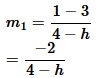is  7x -9y-19 = 0

Since both lines are perpendicular to each other.

Question11. Prove that the line through the point (x1,y1) and parallel to the line Ax + By + C = 0 is A(x − x1) + B(y−y1)=0.

Solution :
Equation of the line parallel to the line Ax + By + C = 0 is …..(i)

Since line (i) passes through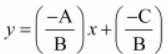, therefore ….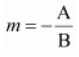.(ii)

Subtracting eq. (ii) from eq. (i), we have

Question 12. Two lines passing through the point (2, 3 ) intersects each other at an angle of 60 If slope of one line is 2, find equation of the other line.

Solution :
Given: Given that the slope of the first line is 2
That is, m1 = 2
Assume that the slope of the other line is m2
The angle between the two lines is 60∘
.

Question13. Find the equation of the right bisector of the line segment joining the points (3, 4) and (-1, 2).

Solution :
The right bisector of a line segment bisects the line segment at an angle 90∘

The end-points of the line segment are given as A(3,4) and B(−1,2)

Now, mid-point of AB=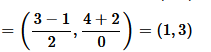⇒ Slope of the required line is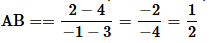Therefore, the required line passes through point (1, 3) having slope -2 .

⇒ Equation of the required line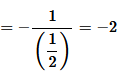The equation of the line passing through (1,3) and having a slope of −2

is,

(y−3) = −2(x−1)

Expand bracket,

y−3 = −2x+2

2x+y = 5

Therefore, the equation of the right bisector of the line segment joining the points (3,4)

and (−1,2)
is 2x+y=5.

Question14. Find the coordinates of the foot of perpendicular from the point (–1, 3) to the line 3x – 4y – 16 = 0.

Solution :
Let Q be the foot of perpendicular drawn from Points (–1, 3) on the line 3x – 4y – 16 = 0.

Slope of the line joining (−1,3) and (a,b) is,

⇒  Equation of a line perpendicular to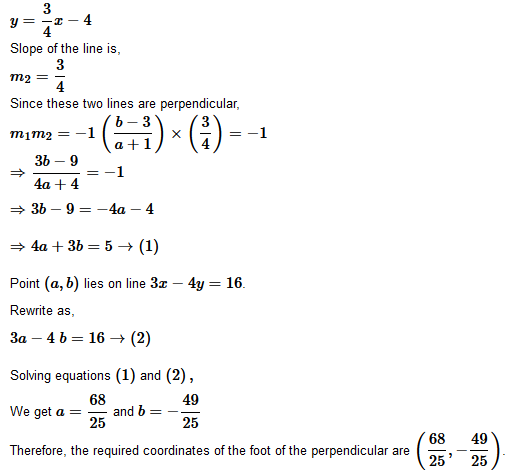Question15. The perpendicular from the origin to the line y = mx + c meets it at the point (–1, 2).  Find the values of m and c.

Solution :
Equation of the line PQ is y = mx + c

The given equation of line is y=mx+c

It is also given that the perpendicular from the origin meets the given line at (−1,2)

.

Then, the line joining the points (0,0)  and (−1,2) is perpendicular to the given line.

Now, slope of the line joining (0,0) and (−1,2)= 2 / −1 = −2

The slope of the given line is m

∴m × −2  = −1

(The two lines are perpendicular )

⇒m = 1/2

Since points (−1,2)  lies on the given line, it satisfies the equation y = mx + c

2 = m(−1) + c

⇒2 = 2 + 12(−1) + c

⇒ c= 2+1 / 2 = 5 / 2

Therefore, the values of m and c are 12 and 52 respectively.

Question 16. If p and q are the lengths of perpendiculars from the origin to the lines cosθ − sin θ = kyx 2cos θ and x sec θ + y cosec θ = k, respectively,  prove that p2+4q2=k2.

Solution :
Length of perpendicular from origin to line is The equation of given lines are,

= xcosθ−ysinθ=kcos2θ→(1)
xsecθ+ycosecθ=k→(2)

And Length of perpendicular from origin to line (d) Ax+By+C=0 from a point (x1,x2)
is

= Compare equation (1)
to the general equation of line that is., Ax+By+C=0,

We get  A = cosθ,B = −sinθ, and C = −kcos2θ

It is given that p is the length of the perpendicular from (0,0) to line (1).

Now, Compare equation (2)
to the general equation of line that is, Ax+By+C = 0

,

We get A = secθ, B = cosecθ
, and C = −k

It is given that q
is the length of the perpendicular from (0,0) to line (2).

Question17. In the triangle ABC with vertices A (2, 3), B (4, –1) and C (1, 2), find the equation and length of altitude from the vertex A.

Solution :
Slope of BC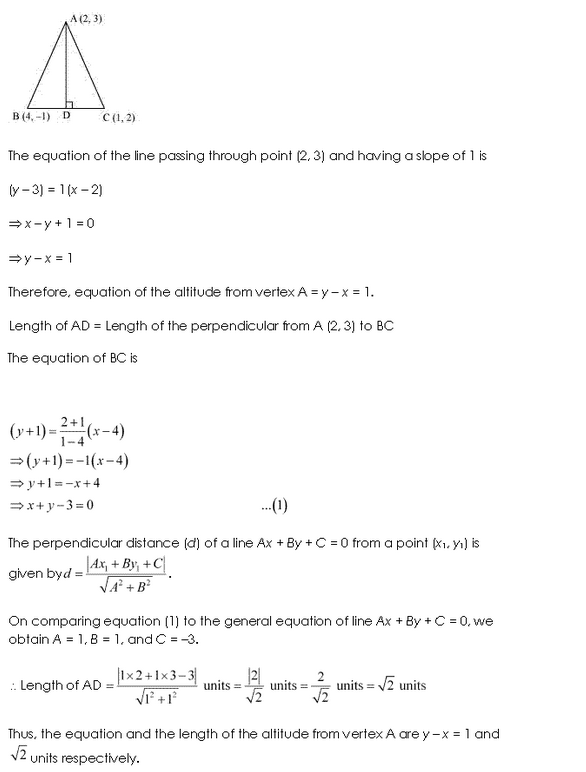Question18. If p is the length of perpendicular from the origin to the line whose intercepts on the axes are a and b, then show that.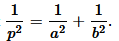Solution :
Given: LineQuestion1. Find the values of k for which the line (k−3)x − (4−k2)y + k2−7k + 6=0

(a) Parallel to the x-axis,

(b) Parallel to the y-axis,

(c) Passing through the origin.

Solution :
Given:
Equation of line (k−3)x − (4−k2)y + k2−7k + 6=0

If the line is parallel to x-axis,

Slope of the line = Slope of the x-axis

(a) If the line parallel to x-axis, then lope of the line = Slope of the x-axis

it can be writen as (k−3)x − (4−k2)y + k2−7k + 6=0

we get

Which is of the form y = mx + c

Here, the slope of the given line

Consider the slope of x-axis =0
= 0

k−3=0

k=3

Therefore, if the given line is parallel to the x
-axis, then the value of k is 3
.

(b) If the line parallel to y-axis, then

The given equation of line is (k−3)x − (4−k2)y + k2−7k + 6=0

Here if the line is parallel to the y-axis, it is vertical and the slope will be undefined.

So, the slope of the given line =

Here, (k−3) / (4−k2) is undefined at k2=4

k2 = 4

⇒k=±2

Therefore, if the given line is parallel to the y
-axis, then the value of k is ±2.

(c) If the line passes through origin then

The given equation of line is (k−3)x − (4−k2)y + k− 7k + 6 = 0

Here, if the line is passing through (0,0)

which is the origin satisfies the given equation

of line,

(k−3)(0) − (4−k2) (0)+k2−7k+6=0

k2−7k+6=0

Separate the terms,

k2−6k−k+6=0

(k−6)(k−1)=0

k=1or 6

Therefore, if the given line is passing through the origin, then the value of k

is either

1or 6

Question2. Find the values of θ and p, if the equation x cos θ +  y sinθ = p is the normal form of the line √3x + y + 2=0.

Solution :
Given:
√3x + y + 2=0.

3x + y = -2

Dividing both sides by,

Question 3. Find the equations of the lines which cut-off intercepts on the axes whose sum and product are 1 and -6 respectively.

Solution :Consider, the intercepts cut by the given lines on the axes are a
and b.

a + b = 1→(1)(1)

ab = − 6 → (2)

Solve both the equations to get

a = 3 and b = −2 or a = −2 and b = 3

We know that the equation of the line whose intercepts on a and b axes is

xa  +  yb  =  1

or

bx  + ay − ab = 0

Case 1:

a = 3
and b = −2

Now, the equation of the line is  −2x + 3y + 6 = 0

That is, 2x − 3y = 6

Case 2:

a=−2
and b=3

Now, the equation of the line is 3x − 2y + 6 = 0

That is, −3x + 2y = 6

Therefore, the required equation of the lines are  2x − 3y = 6
and −3x + 2y = 6.

Question 4. What are the points on the y-axis whose distance from the line x/3 + y/4 = 1 is 4 units?

Solution :
Given: Equation of line (0,b) and let point on x/3 + y/4 = 1 axis be 4

it can be writen  as 4x + 3y -12 = 0 → (1)

compare equation (1) to the perpendicular distance  (d) of a line

4x + 3y -12 = 0  A = 4,B = 3 and C = −12

We know that the perpendicular distance (d) of a line Ax+By+C=0 from (x1,y1) is,

Question 5. Find perpendicular distance from the origin to the line joining the points (cosθ, sin θ) And (cosϕ,sinϕ).

Solution :
Equation of the line joining points
(cosθ, sin θ) and (cosϕ,sinϕ)

y(cosϕ − cosθ) − sinθ(cosϕ − cosθ)

=x(sinϕ − sinθ) − cosθ(sinϕ − sinθ)x (sinθ − sinϕ) − y(cosϕ − cosθ) + cosθ sinϕ − cosθsinθ − sinθ cosϕ  + sinθ cosθ

=0x(sinθ − sinϕ) + y(cosϕ − cosθ) + sin(ϕ−θ)=0

Ax + By + C=0, where A

= sinθ − sinϕ,B= cosϕ − cosθ,  and C=sin(ϕ−θ)

It is known that the perpendicular distance (d) of a line Ax + By + C = 0 from a point (x1, y1) is given by d= |Ax1 + By1 + C| / √A2+√B2.

Therefore, the perpendicular distance (d) of the given line from point (x1, y1) = (0, 0) is

Question 6. Find the equation of the line parallel to y-axis and drawn through the point of intersection of the lines x – 7y + 5 = 0 and 3x + y = 0.

Solution :

The equation of any line parallel to the y-axis is of the form x = a → (1)

The two given lines are x−7y + 5 = 0 → (2)

3x + y = 0 → (3)

Solve equation (2) and (3)
, we get x = −5 / 22 and y = 15 / 22

Thus, (−5 / 22 , 15 /22)
is the point of intersection of lines (2) and (3).

Since line x = a passes through point (−5 / 22,15 / 22),

a = −5 /22

Therefore, the required equation of the line is x = −5 22

Question 7. Find the equation of a line drawn perpendicular to the line x/4 + y/6 = 1 through the point, where it meets the y-axis.

Solution :
Given: Equation of line x/4 + y/6 = 1

Here, the equation of the given line is x 4 + y / 6 = 1

This equation can be written as 3x + 2y − 12 = 0

Rewrite as,

y=−3 / 2x + 6
, which is of the form y = mx + c

Now, Slope of the given line  = −3 / 2

∴Slope of line perpendicular to the given line = −1 / (−3/2 ) = 2 / 3

Let the given line intersect the y-axis at (0,y)

.

Substitute x with 0 in the equation of the given line,

y / 6 = 1

⇒ y = 6

∴ The given line intersects the y-axis at (0,6)

The equation of the line that has a slope of 23 and passes through point (0,6) is,(y−6)=23(x−0) Cross multiply and expand brackets,

3y − 18 = 2x

2x − 3y + 18 = 0

Therefore, the required equation of the line is 2x − 3y + 18 = 0
.

Get 30% off your first purchase!

X
error: Content is protected !!
Scroll to Top